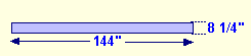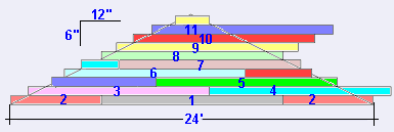# Calculate the amount of Fiber Cement Siding needed to cover a GableThe square footage of the Gable (green area) = 6 * 24 / 2 = 72 sq. ft.The Fibercement board we are using here is 144 " long and 8 1/4" wide. We are going to install it with a 1 1/4" overlap giving it an exposed face of 7"#### Calculate the lineal area factor (llf) of your siding.

To calculate the llf take the exposed face value of your siding and 12".
Divide the 12" by the llf of 7"
Exposed face value of 7"
12 / 7 = 1.743
Multiply the square footage of the Gable area of 72 sq. by this amount, and this will give you the lineal feet of siding needed to cover the gable
72 * 1.743 = 123.43 sq. ft.
Divide this by the board length of your siding
123.43 / 12 = 10.29
Ideally, without any waste, it would take 10 and one third lengths of boards to cover this Gable.Placing the boards on the gable and counting the full boards used, we come to 11 full boards to cover this Gable. To cover this Gable there is approx. 10% waste.